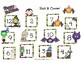# Halloween Roll & Cover 1-6 & 2-12Subject
Resource Type
File Type

PDF

(4 MB)
Product Rating
Standards
• Product Description
• StandardsNEW

This could be used at a Halloween party with dice or as an early finisher math game.

Add and subtract within 20, demonstrating fluency for addition and subtraction within 10. Use strategies such as counting on; making ten (e.g., 8 + 6 = 8 + 2 + 4 = 10 + 4 = 14); decomposing a number leading to a ten (e.g., 13 - 4 = 13 - 3 - 1 = 10 - 1 = 9); using the relationship between addition and subtraction (e.g., knowing that 8 + 4 = 12, one knows 12 - 8 = 4); and creating equivalent but easier or known sums (e.g., adding 6 + 7 by creating the known equivalent 6 + 6 + 1 = 12 + 1 = 13).
Understand that the last number name said tells the number of objects counted. The number of objects is the same regardless of their arrangement or the order in which they were counted.
When counting objects, say the number names in the standard order, pairing each object with one and only one number name and each number name with one and only one object.
Count forward beginning from a given number within the known sequence (instead of having to begin at 1).
Total Pages
N/A
N/A
Teaching Duration
N/A
Report this Resource to TpT
Reported resources will be reviewed by our team. Report this resource to let us know if this resource violates TpT’s content guidelines.